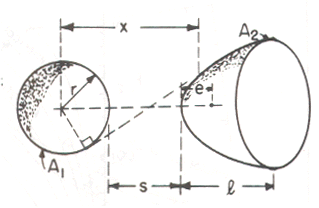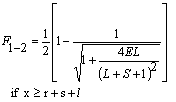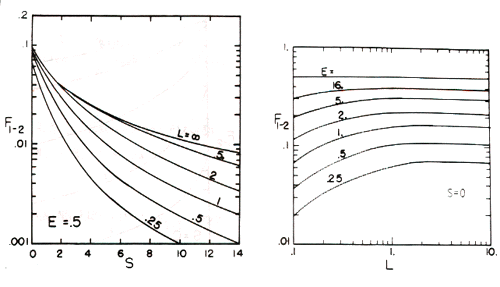# SECTION C Factors From Finite Areas to Finite Areas

## C-141: Sphere to coaxial paraboloid; e is distance from focus to vertex of paraboloid, x is the distance between the sphere center and a tangent point on the paraboloid in a plane containing the axis.Definitions:

 S = s/r; L = l/r E = e/r

Governing Equation:To download a copy of this equation in MS Word 97 Format, click HERE.